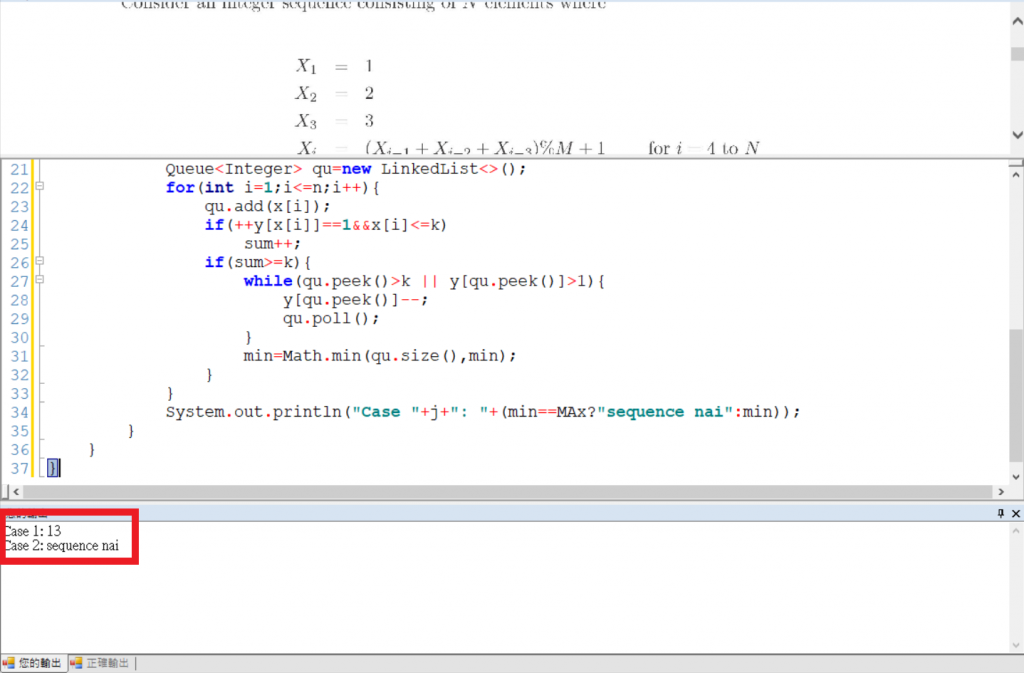#DAY 29
0

## [iT鐵人賽Day29]練習題(8)

{1,2,3,7,1,12,9,11,9,6,3,7,5,4,5,3,1,10,3,3}

``````import java.util.*;
class main{
static int MAx=Integer.MAX_VALUE;
public static void main(String[] args){
Scanner sc=new Scanner(System.in);
int t=sc.nextInt();
for(int j=1;j<=t;j++){
int n=sc.nextInt(),m=sc.nextInt(),k=sc.nextInt();
int[] x=new int[n+1];
x=1;
x=2;
x=3;
for(int i=4;i<=n;i++){
x[i]=(x[i-1]+x[i-2]+x[i-3])%m+1;
}
int[] y=new int;
int sum=0,min=MAx;
for(int i=1;i<=n;i++){
if(++y[x[i]]==1&&x[i]<=k)
sum++;
if(sum>=k){
while(qu.peek()>k || y[qu.peek()]>1){
y[qu.peek()]--;
qu.poll();
}
min=Math.min(qu.size(),min);
}
}
System.out.println("Case "+j+": "+(min==MAx?"sequence nai":min));
}
}
}
``````